# Array Lists Array Lists and arrays n n

• Slides: 14Array. Lists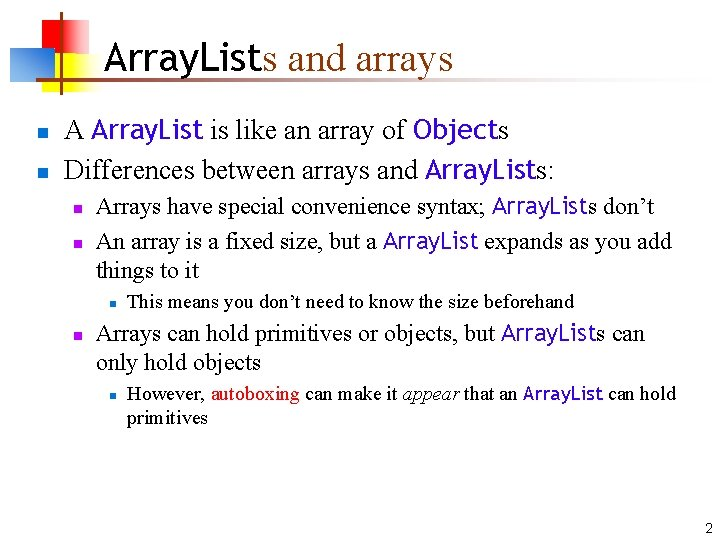Array. Lists and arrays n n A Array. List is like an array of Objects Differences between arrays and Array. Lists: n n Arrays have special convenience syntax; Array. Lists don’t An array is a fixed size, but a Array. List expands as you add things to it n n This means you don’t need to know the size beforehand Arrays can hold primitives or objects, but Array. Lists can only hold objects n However, autoboxing can make it appear that an Array. List can hold primitives 2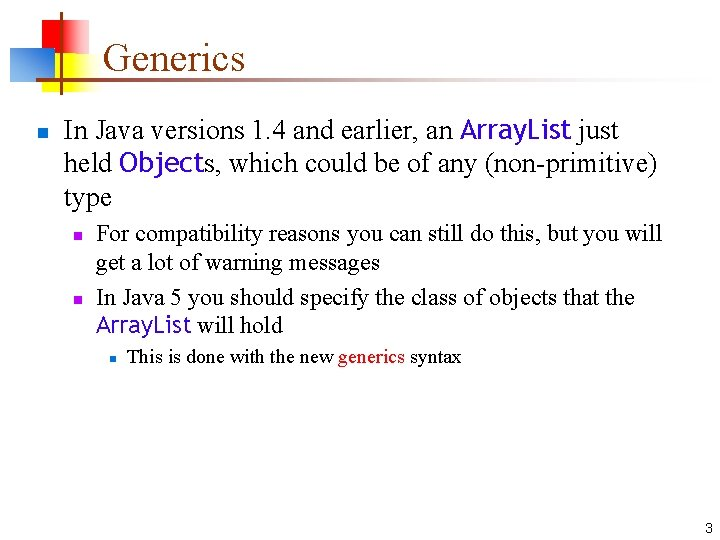Generics n In Java versions 1. 4 and earlier, an Array. List just held Objects, which could be of any (non-primitive) type n n For compatibility reasons you can still do this, but you will get a lot of warning messages In Java 5 you should specify the class of objects that the Array. List will hold n This is done with the new generics syntax 3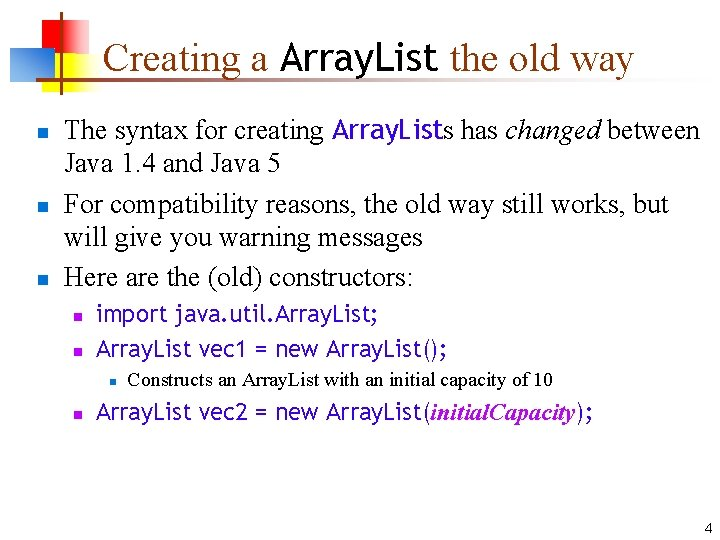Creating a Array. List the old way n n n The syntax for creating Array. Lists has changed between Java 1. 4 and Java 5 For compatibility reasons, the old way still works, but will give you warning messages Here are the (old) constructors: n n import java. util. Array. List; Array. List vec 1 = new Array. List(); n n Constructs an Array. List with an initial capacity of 10 Array. List vec 2 = new Array. List(initial. Capacity); 4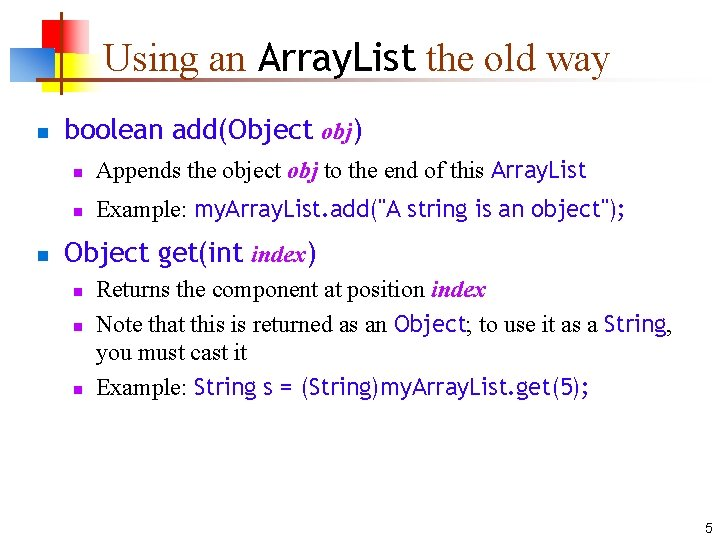Using an Array. List the old way n n boolean add(Object obj) n Appends the object obj to the end of this Array. List n Example: my. Array. List. add("A string is an object"); Object get(int index) n n n Returns the component at position index Note that this is returned as an Object; to use it as a String, you must cast it Example: String s = (String)my. Array. List. get(5); 5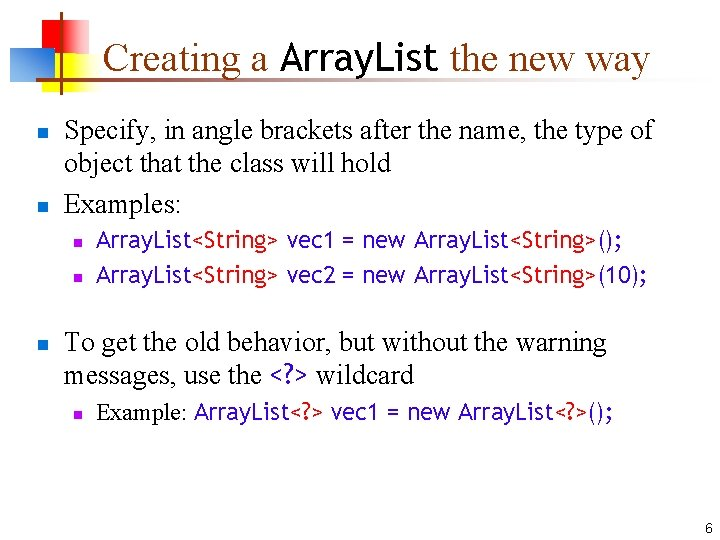Creating a Array. List the new way n n Specify, in angle brackets after the name, the type of object that the class will hold Examples: n n n Array. List<String> vec 1 = new Array. List<String>(); Array. List<String> vec 2 = new Array. List<String>(10); To get the old behavior, but without the warning messages, use the <? > wildcard n Example: Array. List<? > vec 1 = new Array. List<? >(); 6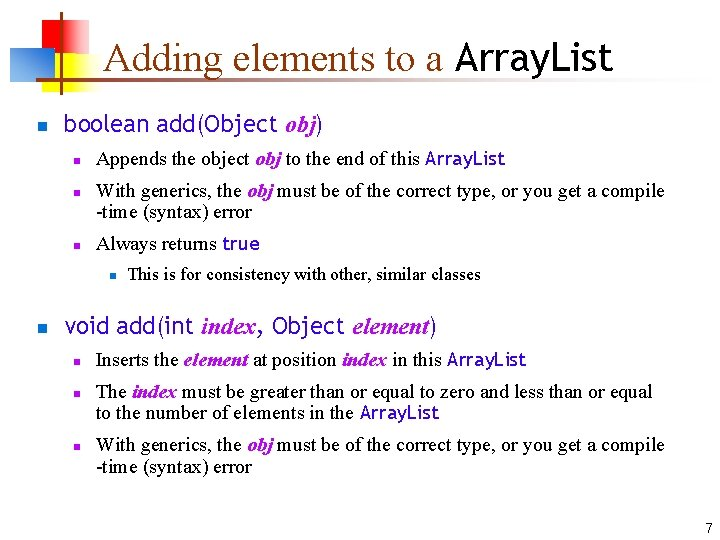Adding elements to a Array. List n boolean add(Object obj) n n n Appends the object obj to the end of this Array. List With generics, the obj must be of the correct type, or you get a compile -time (syntax) error Always returns true n n This is for consistency with other, similar classes void add(int index, Object element) n n n Inserts the element at position index in this Array. List The index must be greater than or equal to zero and less than or equal to the number of elements in the Array. List With generics, the obj must be of the correct type, or you get a compile -time (syntax) error 7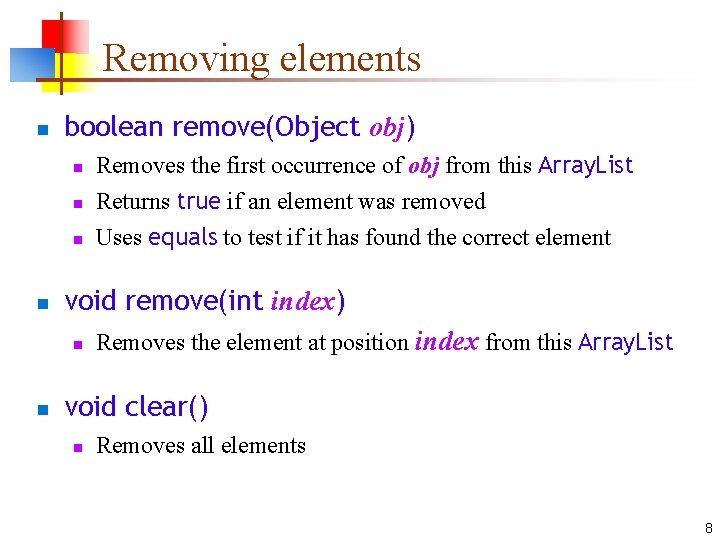Removing elements n boolean remove(Object obj) n n void remove(int index) n n Removes the first occurrence of obj from this Array. List Returns true if an element was removed Uses equals to test if it has found the correct element Removes the element at position index from this Array. List void clear() n Removes all elements 8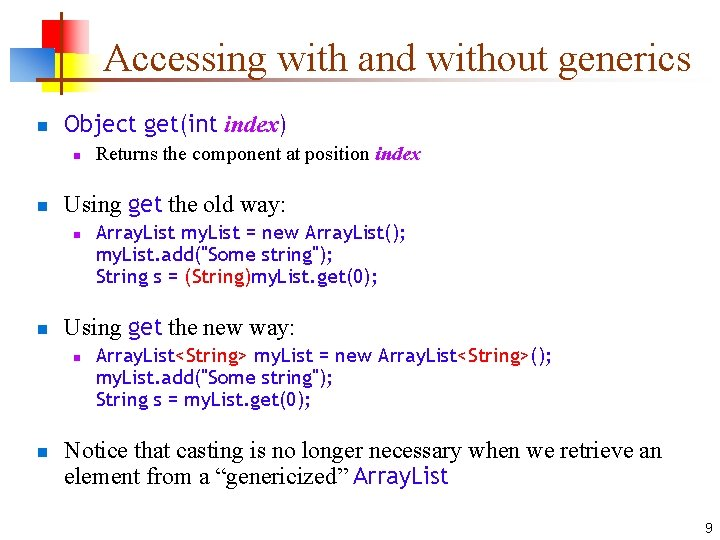Accessing with and without generics n Object get(int index) n n Using get the old way: n n Array. List my. List = new Array. List(); my. List. add("Some string"); String s = (String)my. List. get(0); Using get the new way: n n Returns the component at position index Array. List<String> my. List = new Array. List<String>(); my. List. add("Some string"); String s = my. List. get(0); Notice that casting is no longer necessary when we retrieve an element from a “genericized” Array. List 9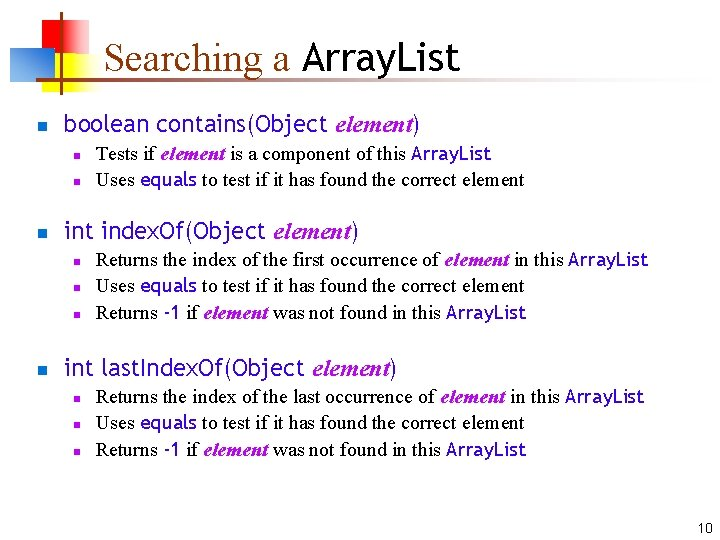Searching a Array. List n boolean contains(Object element) n n n int index. Of(Object element) n n Tests if element is a component of this Array. List Uses equals to test if it has found the correct element Returns the index of the first occurrence of element in this Array. List Uses equals to test if it has found the correct element Returns -1 if element was not found in this Array. List int last. Index. Of(Object element) n n n Returns the index of the last occurrence of element in this Array. List Uses equals to test if it has found the correct element Returns -1 if element was not found in this Array. List 10Getting information n boolean is. Empty() n n int size() n n Returns true if this Array. List has no elements Returns the number of elements currently in this Array. List Object[ ] to. Array() n Returns an array containing all the elements of this Array. List in the correct order 11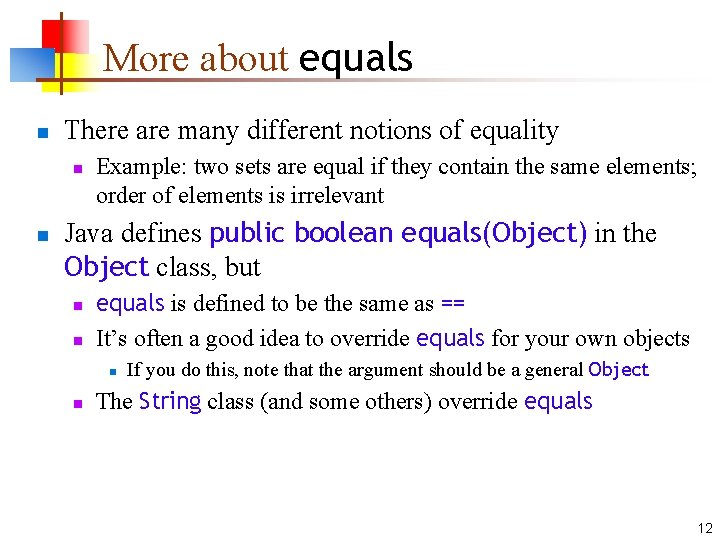More about equals n There are many different notions of equality n n Example: two sets are equal if they contain the same elements; order of elements is irrelevant Java defines public boolean equals(Object) in the Object class, but n n equals is defined to be the same as == It’s often a good idea to override equals for your own objects n n If you do this, note that the argument should be a general Object The String class (and some others) override equals 12Conclusion n n A Array. List is like an array of Objects The advantage of a Array. List is that you don’t need to know beforehand how big to make it The disadvantage of a Array. List is that you can’t use the special syntax for arrays You should never use an array that you hope is “big enough”—use a Array. List instead 13The End “Where a calculator on the ENIAC is equipped with 18 000 vacuum tubes and weighs 30 tons, computers of the future may have only 1 000 vacuum tubes and perhaps weigh 1½ tons. ” --Popular Mechanics, March 1949 14